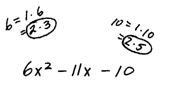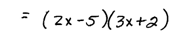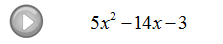## Algebra

### Factoring Trinomials of the Form ax^2 + bx + c

So far we have looked at trinomials with leading coefficients of 1. Now we will see how the process is changed when the leading coefficients are something other than 1.

Factor:Step 1: Look at the factors of the first and last terms.Step 2: Choose the factors where the multiples add to the middle term.Step 3: Determine the signs using the product of the inner and outer terms.Step 4. Check by multiplying.

As you can see there are many more combinations to consider when the leading coefficient is not 1. These take time but become routine with practice.

Factor the trinomials.